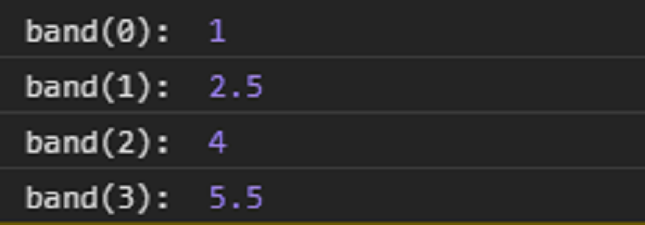# D3.js bandScale band() Function

• Last Updated : 23 Sep, 2020

The band() function in d3.js library is used to return a start of the band from the range corresponding to the given input domain.

Syntax:

Hey geek! The constant emerging technologies in the world of web development always keeps the excitement for this subject through the roof. But before you tackle the big projects, we suggest you start by learning the basics. Kickstart your web development journey by learning JS concepts with our JavaScript Course. Now at it's lowest price ever!

`band(value);`

Parameters: This function takes a single parameter as given above and described below.

• Value: This accepts a value from the specified domain.

Return Value: This function returns a value from the specified range.

Example: The following example demonstrates the above function.

## HTML

 ```<``html` `lang``=``"en"``>`` ` `<``head``>``    ``<``meta` `charset``=``"UTF-8"` `/>``    ``<``meta` `name``=``"viewport"` `path1tent``=``        ``"width=device-width, initial-scale = 1.0"` `/>``    ``<``script` `src``=``"https://d3js.org/d3.v4.min.js"``>``    `````` ` `<``body``>``    ``<``script``>``        ``// Create the band scale with ``        ``// specified domain and range.``        ``var band = d3.scaleBand()``            ``.domain([0, 1, 2, 3, 4, 5])``            ``.range([1, 10]);``             ` `        ``// Discrete values are automatically ``        ``// created by the band function``        ``// band of the range is [1, 10]``        ``console.log("band(0): ", band(0));``        ``console.log("band(1): ", band(1));``        ``console.log("band(2): ", band(2));``        ``console.log("band(3): ", band(3));``    `````` ` ``

Output:My Personal Notes arrow_drop_up